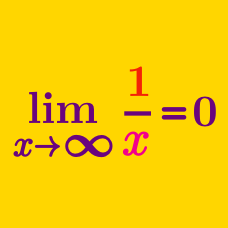Calculus

# Limits of Functions: Level 5 Challenges

$\large \lim_{n\rightarrow \infty} \, \sqrt[ \large n^{2}+n]{\binom{n}{0}\binom{n}{1}\binom{n}{2}\cdots \binom{n}{n}}$

Find the value of the closed form of the above limit to 3 decimal places.

Let $(s_n)_{n=0}^{\infty}$ be a sequence of real numbers defined as follows:

$s_0 = 2; s_{n+1} = \sqrt{2-\sqrt{4-s_n^2}}$ for $n \ge 0$.

To the nearest hundredth, find the value of $\displaystyle\lim_{n \to \infty} 2^n s_n$.

In other words, to what value does the following sequence converge:$2^3 s_3 = 8\sqrt{2-\sqrt{2+\sqrt{2}}}$$2^4 s_4 = 16\sqrt{2-\sqrt{2+\sqrt{2+\sqrt{2}}}}$$2^5 s_5 = 32\sqrt{2-\sqrt{2+\sqrt{2+\sqrt{2+\sqrt{2}}}}}$and so on...

$\large \lim_{n\to\infty} \sqrt[n^2]{{n \choose1}{n \choose 2}\cdots{n \choose n}}$

Find the closed form of the limit above to 3 decimal places.

Notation: $\displaystyle {n \choose k} = \dfrac {n!}{k!(n-k)!}$ denotes the binomial coefficient.

For $a=\dfrac1{16}$, consider the (finite) power tower,

$\Large x_n=\underbrace{a^{a^{\cdot^{\cdot^{a^a}}}}}_{2n \; a\text{'s}}$

For example, $x_1=a^a$ and $x_2=a^{a^{a^a}}$.

Find $\displaystyle \lim_{n\to\infty}x_n$, to three significant figures.

Bonus What happens if we consider a power tower with an odd number of $a$'s?

\begin{aligned} f(x) &= \begin{cases} 0 &\text{ if } x \text{ is irrational} \\ 1 &\text{ if } x \text{ is rational} \end{cases} \\ g(x) &= \begin{cases} 0 &\text{ if } x \text{ is irrational} \\ \frac1q &\text{ if } x =\frac{p}{q}, \text{ where } p \text{ and } q \text{ are coprime nonnegative integers} \end{cases} \end{aligned}

Let $f(x)$ and $g(x)$ be two functions defined on $[0,1]$ by the formulas as described above.

For which $a \in (0,1)$ does the (deleted) $\lim\limits_{x\to a} f(x)$ exist?

For which $b \in (0,1)$ does the (deleted) $\lim\limits_{x\to b} g(x)$ exist?

×